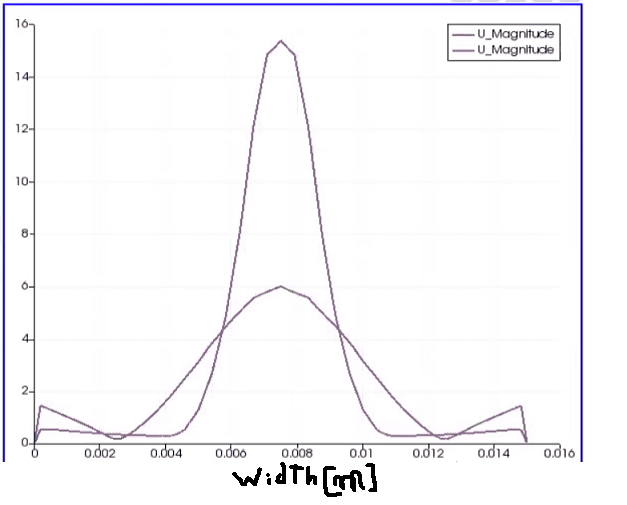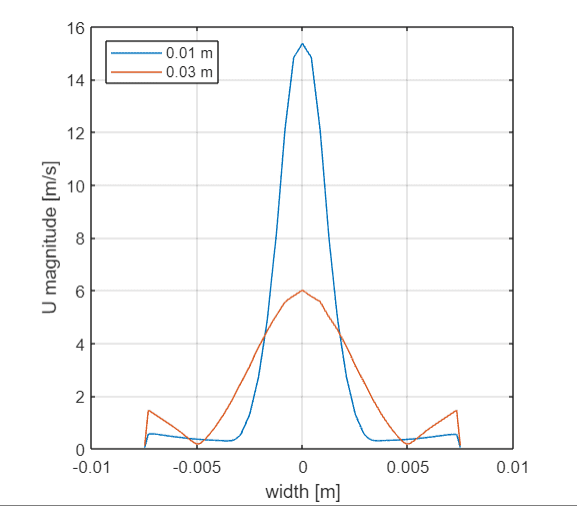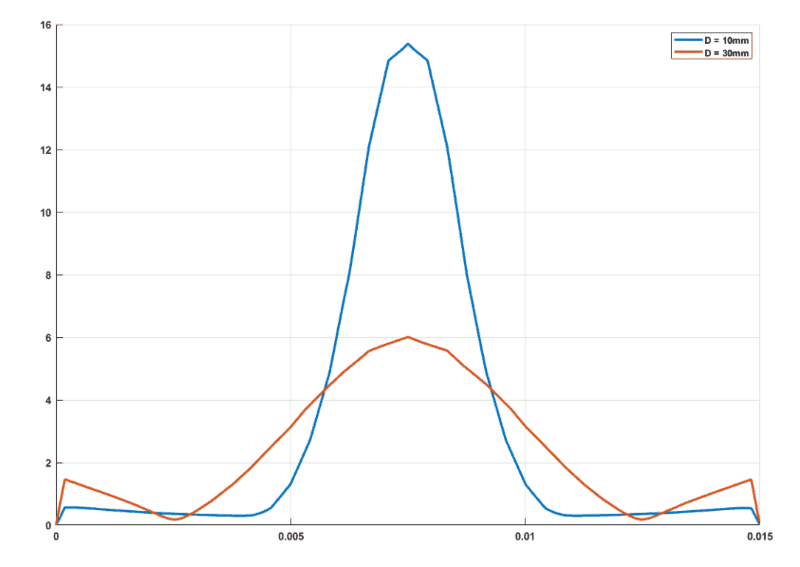# How to plot data in MATLAB?

• MATLAB
• JD_PM
In summary, the conversation discusses studying a flow through an orifice and creating two tables for different downstream distances. The data for each table is in CSV format and will be used to plot the speed distribution over the vertical width profile for each distance. The algorithm to do this involves optimizing the given table, reading the velocity data, computing the speed, and storing the speed and width values. The code for this process is provided, but the issue is automating it for 1000 rows. A possible solution using structs is suggested, and a code that has been developed so far is shared. f

#### JD_PM

TL;DR Summary
I am aimed at overlapping the plots for the speed distribution over the vertical width profile (which is 0.015 m long; highlighted in red) for two downstream, horizontal distances [w.r.t. the orifice]: 0.01 m and 0.03 m.

How to modify my code such that it swaps all row data?
I am studying a flow going through an orifice.

I am aimed at overlapping the plots for the speed distribution over the vertical width profile (which is 0.015 m long; highlighted in red) for two downstream, horizontal distances [w.r.t. the orifice]: 0.01 m and 0.03 m.The result should look as follows (the highest peak corresponds to 0.01m width profile)I have the data for both downstream distances (in csv format, here you can find it https://drive.google.com/drive/folders/1CgeDuCahVZvLXXkNkGWlPg8lSGyurmI3).

Let me create two tables, first related to 0.01m data and second to 0.03 m. For the sake of simplicity I only include three row data. The columns labeled - contain irrelevant data for this particular problem.

0.01m data

 U0 U1 U2 - - X Y Z -0.044326 3.0829e-05 0.0016394 1 1.5e-05 0.01 0 -0.007485 -0.088655 6.1659e-05 0.003279 1 3e-05 0.01 0 -0.00747 -0.13298 9.2489e-05 0.0049184 1 4.5e-05 0.01 0 -0.007455

0.03m data

 U0 U1 U2 - - X Y Z -0.11972 -0.00036133 -0.0012355 1 1.5e-05 0.03 0 -0.007485 -0.23945 -0.00072269 -0.002471 1 3e-05 0.03 0 -0.00747 -0.35918 -0.001084 -0.0037066 1 5e-05 0.03 0 -0.007455

Let's draw our attention to how to code this in MATLAB. The algorithm I have in mind is:

0) Optimize the given table: eliminate first and last rows (the velocity there is zero due to no-slip condition on the walls)

1) Read the velocity data from the csv table and compute the speed ##U = \sqrt{U_0^2 + U_1^2 + U_2^2}## for each data row.

2) Store the speed and width values in variables.

3) Repeat 2) and 3) for the other csv file.

I managed to write a code that works for the three-rows-of-data tables above

Matlab:
Ai = readtable("sampleData10mm.csv"); %step 0)
A = Ai(2:1000,:); %step 0)
fComr1 = A{1:1,"U_0"}; %step 1)
sComr1 = A{1:1,"U_1"}; %step 1)
tComr1 = A{1:1,"U_2"}; %step 1)
fComr2 = A{2:2,"U_0"}; %step 1)
sComr2 = A{2:2,"U_1"}; %step 1)
tComr2 = A{2:2,"U_2"}; %step 1)
fComr3 = A{3:3,"U_0"}; %step 1)
sComr3 = A{3:3,"U_1"}; %step 1)
tComr3 = A{3:3,"U_2"}; %step 1)

Ur1 = sqrt(fComr1^2 + sComr1^2 + tComr1^2); %step 1)
Ur2 = sqrt(fComr2^2 + sComr2^2 + tComr2^2); %step 1)
Ur3 = sqrt(fComr3^2 + sComr3^2 + tComr3^2); %step 1)

speed = [0;Ur1;Ur2;Ur3]; %step 2)
width = [0;A{1:1,"Points_2"}+0.0075;A{2:2,"Points_2"}+0.0075;A{3:3,"Points_2"}+0.0075]; %step 2); Note I added +0.0075 because we want to have data from 0 to 0.016 mm in the x axis

B = Bi(2:1000,:); %step 3)
fComr1_30mm = B{1:1,"U_0"};  %step 3)
sComr1_30mm = B{1:1,"U_1"};  %step 3)
tComr1_30mm = B{1:1,"U_2"};  %step 3)
fComr2_30mm = B{2:2,"U_0"};  %step 3)
sComr2_30mm = B{2:2,"U_1"};  %step 3)
tComr2_30mm = B{2:2,"U_2"};  %step 3)
fComr3_30mm = B{3:3,"U_0"};  %step 3)
sComr3_30mm = B{3:3,"U_1"};  %step 3)
tComr3_30mm = B{3:3,"U_2"};  %step 3)
Ur1_30mm = sqrt(fComr1_30mm^2 + sComr1_30mm^2 + tComr1_30mm^2); %step 3)
Ur2_30mm = sqrt(fComr2_30mm^2 + sComr2_30mm^2 + tComr2_30mm^2); %step 3)
Ur3_30mm = sqrt(fComr3_30mm^2 + sComr3_30mm^2 + tComr3_30mm^2); %step 3)
speed_30mm = [0;Ur1_30mm;Ur2_30mm;Ur3_30mm]; %step 3)
width_30mm = [0;B{1:1,"Points_2"}+0.0075;B{2:2,"Points_2"}+0.0075;B{3:3,"Points_2"}+0.0075]; %step 3)

figure %step 4)
plot(width,width_30mm,speed,speed_30mm) %step 4)
legend({'0.01 m', '0.03 m'},'Location','northwest') %step 4)
xlabel('width [m]') %step 4)
ylabel('U magnitude [m/s]') %step 4)
axis square; %step 4)
grid on; %step 4)

The issue I have is that I do not see how to write a code that does it for the 1000 rows. I guess there has to be a way of automatizing the whole process...

I am quite sure the code can be written more efficiently (it's my first MATLAB code ever!), so please feel to add suggestions :)

Thank you!PS: Shout out to the Moderation Staff for asking me to write more details!this looks pretty straight-forward. i'll give you an idea, maybe not the whole clean code, but enough to point you to a viable solution

i use structs fairly often for things like this, though probably not the most efficient.

Matlab:
data=struct(); %creates struct
for i=1:length(A);   %from 1 to size of table
data(1).u0(i)=A{i:i,"U_0"};    %speeds at first line
data(1).u1(i)=A{i:i,"U_1"};
data(1).u2(i)=A{i:i,"U_2"};
data(2).u0(i)=B{i:i,"U_0"};    %speeds at second line
data(2).u1(i)=B{i:i,"U_1"};
data(2).u2(i)=B{i:i,"U_2"};
data(1).mag=(data(1).u0(i)^2+data(1).u1(i)^2+data(1).u2(i)^2)^.5   %calculates magnitude for every point
data(2).mag=(data(2).u0(i)^2+data(2).u1(i)^2+data(2).u2(i)^2)^.5

data(1).z(i)=A{i:i,"Z"};   %gathers the z points into a vector

end
plot(data(1).z, data(1).mag);
hold on
plot(data(1).z, data(2).mag);

edit:
--------
Honestly, it's pretty quick and easy to do this all in excel...

Last edited by a moderator:
•JD_PM
Nice, thank you! I am not familiar with structs so I will definitely learn from it.

Let me share the code I got so far

Matlab:
clc
close all
clear all
warning('OFF', 'MATLAB:table:ModifiedAndSavedVarnames')

%step 0
A = Ai(2:1000,:);
B = Bi(2:1000,:);

%step 1
fCom_10mm = A{:,"U_0"};
sCom_10mm = A{:,"U_1"};
tCom_10mm = A{:,"U_2"};
width_10mm = A{:,"Points_2"};
U_10mm = sqrt(fCom_10mm.^2 + sCom_10mm.^2 + tCom_10mm.^2);
%refined_width_10mm = [0;A(:,"Points_2")+0.0075];
%refined_width_10mm = A{:,"Points_2"+0.0075};

%step 2
fCom_30mm = B{:,"U_0"};
sCom_30mm = B{:,"U_1"};
tCom_30mm = B{:,"U_2"};
width_30mm = B{:,"Points_2"};
U_30mm = sqrt(fCom_30mm.^2 + sCom_30mm.^2 + tCom_30mm.^2);
%refined_width_30mm = [0;B(:,"Points_2")+0.0075];
%refined_width_30mm = B{:,"Points_2" + 0.0075};

%step 3
figure
plot(width_10mm,U_10mm,width_30mm,U_30mm)
%plot(refined_width_10mm,U_10mm,refined_width_30mm,U_30mm)
legend({'0.01 m', '0.03 m'},'Location','northwest')
xlabel('width [m]')
ylabel('U magnitude [m/s]')
axis square;
grid on;

Which yieldsI am almost there. I am working on how to get the zero at the x-axis y-axis intersection: